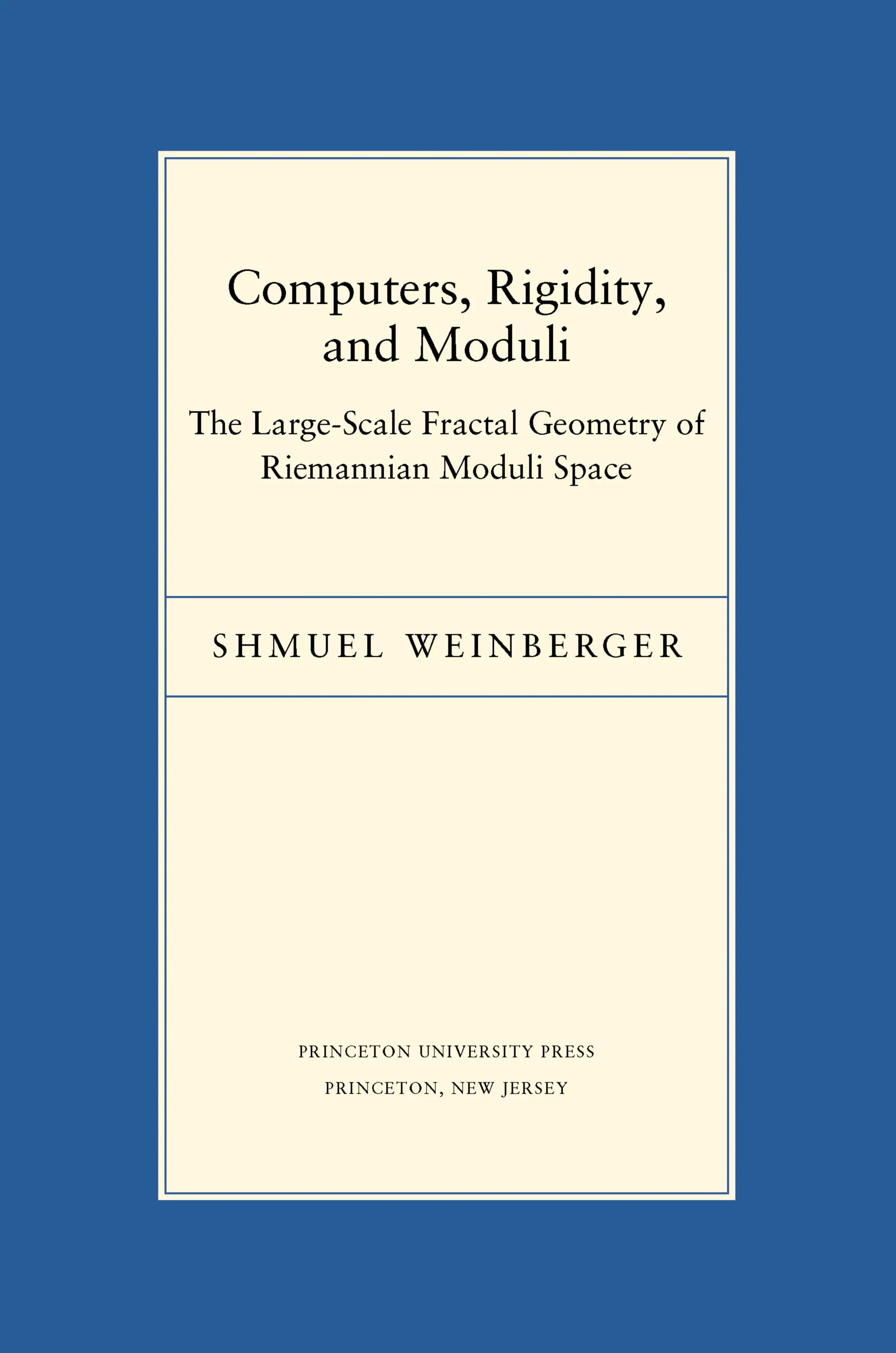#### Computers, Rigidity, and Moduli

Shmuel Weinberger

# Computers, Rigidity, and Moduli: The Large-Scale Fractal Geometry of Riemannian Moduli Space### Hardcover

Price:
\$80.00 / £66.00
ISBN:
Published:
12/19/2004
2005
Pages:
192
Size:
6 x 9.25 in.
Illus:
9 line illus.

This book is the first to present a new area of mathematical research that combines topology, geometry, and logic. Shmuel Weinberger seeks to explain and illustrate the implications of the general principle, first emphasized by Alex Nabutovsky, that logical complexity engenders geometric complexity. He provides applications to the problem of closed geodesics, the theory of submanifolds, and the structure of the moduli space of isometry classes of Riemannian metrics with curvature bounds on a given manifold. Ultimately, geometric complexity of a moduli space forces functions defined on that space to have many critical points, and new results about the existence of extrema or equilibria follow.

The main sort of algorithmic problem that arises is recognition: is the presented object equivalent to some standard one? If it is difficult to determine whether the problem is solvable, then the original object has doppelgängers — that is, other objects that are extremely difficult to distinguish from it.

Many new questions emerge about the algorithmic nature of known geometric theorems, about “dichotomy problems,” and about the metric entropy of moduli space. Weinberger studies them using tools from group theory, computability, differential geometry, and topology, all of which he explains before use. Since several examples are worked out, the overarching principles are set in a clear relief that goes beyond the details of any one problem.